×#### Thank you for registering.

One of our academic counsellors will contact you within 1 working day.

Click to Chat

1800-1023-196

+91-120-4616500

CART 0

• 0

MY CART (5)

Use Coupon: CART20 and get 20% off on all online Study Material

ITEM
DETAILS
MRP
DISCOUNT
FINAL PRICE
Total Price: Rs.

There are no items in this cart.
Continue Shopping```Integral Calculus

Table of Content

Indefinite Integral

Definite Integral

Properties of Definite IntegralIntegral Calculus is the part of calculus that deals with integration and its application in the solution of differential equations and in determining areas or volumes etc.It involves problems like solution of area and volume. In the figure given above, integral calculus may be used to find out the area between the two curves F1 and F2.

It would not be an exaggeration if we call Calculus to be the castle of Mathematics and its most important part is the integral calculus. The topic is quite scoring and has a good weightage in exams like IIT JEE, AIEEE etc. but it can be mastered only through constant practice.

Definite integrals and Indefinite integrals are the major tools which are used for applications under the topic of Area. Calculation of area is considered a hard topic but since it fetches many questions in JEE so it must be given due importance.

Indefinite Integral

An integral of the form  i.e. without upper and lower limits is called an indefinite integral. Indefinite integrals are often written aswhere c is an arbitrary constant. Indefinite integrals are also called Antiderivatives.

Note: The constant of integration is very important. In fact, the graphs of Antiderivatives of a function are vertical translations of each other, the location of each graph depending on the value of c. Consider the following example:

The function F(x) = x3/3 is an antiderivative of f(x) = x2. As the derivative of constant is zero, x2 will have an infinite number of antiderivatives; such as (x3/3) + 0, (x3/3) + 7, (x3/3) − 42, (x3/3) + 293 etc. Thus, all the antiderivatives of x2 can be obtained by changing the value of C in F(x) = (x3/3) +C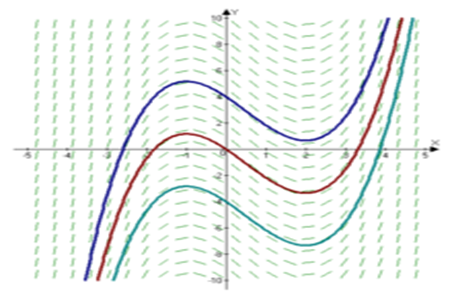Illustration:

Solve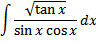Solution:=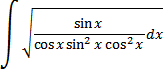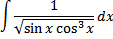=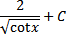=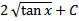Definite Integral

Given a function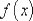that is continuous on the interval [a,b] we divide the interval into n subintervals of equal width,, and from each interval choose a point x1*  Then the definite integral of f(x) from a to b is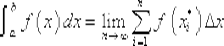Properties of Definite Integral

We list below various important rules that form the basis of solving numerical in definite integral:

(1) .The limits on any definite integral can be interchanged. You just need to add a minus sign on the integral while doing so.

(2) .When the upper and lower limits coincide, the value of the integral is zero.

(3), where c is any number.

A constant can be taken out of the integral sign in case of both definite and indefinite integral.

(4)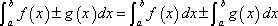.

A definite integral can be broken into parts across a sum or difference.

(5)where c is any number.

This property tells us how to integrate a function over adjacent intervals, [a,c] and [c,b]. Note that it is not necessary for c to be between a and b.

(6)This property shows that as long as the function and limits are same, the variable used for integration does not make any difference.

Illustration 1: Let f(x) = x/(1+xn)1/n for n ≥ 2 and g(x) = (fofofofof… n times) (x). Then find the value of ∫xn-2 g(x) dx.

Solution: The value of f(x) is given to be f(x) = x/(1+xn)1/n

Then ff(x) = f(x)/(1 + f(x)n)1/n

= x/(1+2xn)1/n

So, fff(x) = x/(1+3xn)1/n

Hence, g(x) = (fofof…..n times)(x) = x/(1+nxn)1/n

Hence, I = ∫xn-2 g(x) dx

= ∫ xn-1dx/(1 + nxn)1/n

= 1/n2 ∫ n2xn-1dx/(1 + nxn)1/n

= 1/n2 ∫ [d/dx (1 + nxn)] / (1 + nxn)1/n . dx

Hence, I = [(1+ nxn)1-1/n ]/ n(n-1) + K.

Illustration 2: Evaluate the given expression

∫ dx/ [ x2(x4 + 1)]3/4

Solution: Let I = ∫ dx/ [x2(x4 + 1)]3/4

= ∫ dx/ x2x3(1+x-4)3/4

= ∫ dx/ x5(1+x-4)

Put 1+x-4 = z

Then -4x-5 dx = dz

Hence, I = ∫ -dz/4z3/4

= -1/4 ∫ dz/z3/4

= -z1/4 + c

Illustration 3: Evaluate ∫ (x +1)/ x(1+xex)2 dx.

Solution: Let I = ∫ (x +1)/ x(1+xex)2 dx

= ∫ ex (x +1)/ xex (1+xex)2 dx

Put (1+xex) = t, then (ex+xex) dx = dt

Hence, I = ∫ dt/ (t-1)t2 = ∫[1/(t-1) – 1/t – 1/t2] dt

= log |t-1| - log |t| +1/t + c

= log | [(t-1)/t] | +1/t + c

= log | xex/ (1+xex)| + 1/ (1+xex) + c

Watch this Video for more reference

Most of the students are weak in Integral calculus but this weakness must be converted into strength with regular practice. All the high rank holders in JEE have their expertise in Integral calculus.

To read more, Buy study materials of Indefinite integral comprising study notes, revision notes, video lectures, previous year solved questions etc. Also browse for more study materials on Mathematics here.
```### Course Features

• 731 Video Lectures
• Revision Notes
• Previous Year Papers
• Mind Map
• Study Planner
• NCERT Solutions
• Discussion Forum
• Test paper with Video Solution